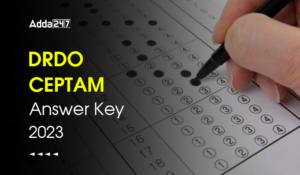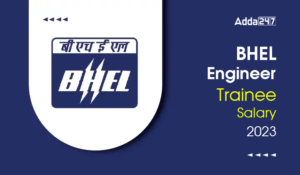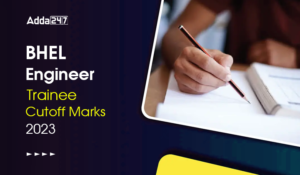Engineering Jobs   »   Mechincal Engineering quizs   »   UPPSC Lecturer Recruitment 2021, UPPSC Lecturer...

# UPPSC Lec’21 ME: Daily Practices Quiz. 07-Dec-2021

Quiz: Mechanical Engineering
Exam: UPPSC Lecturer
Topic: Fluid Mechanics

Each question carries 3 marks
Negative marking: 1 mark
Time: 6 Minutes

Q1. The discharge through a venturimeter is given as (with usual notation):Q2. Bluff body surface…………………
(a) Is smooth so that friction can be neglected
(b) Coincides with stream lines
(c) Does not coincide with stream lines
(d) Perpendicular to stream lines

Q3. The head loss in a pipe of diameter d, carrying oil at a flow rate Q over a distance L is h. This pipe is replaced by another with half the diameter, all other things remaining same, the head loss in this case would be
(a) 0.5 h
(b) 2.0 h
(c) 8.0 h
(d) 32.0 h

Q4. As the depth of immersion of a vertical plane surface increase, the location of centre of pressure
(a) Falls closer to the centre of gravity of the area
(b) Moves away from the centre of gravity of the area
(c) Ultimately coincides with the centre of gravity of the area
(d) None of these

Q5. One stokes is equal to
(a) 1 cm²/s
(b) 1 m²/s
(c) 1 mm²/s
(d) 10 m²/s

Q6. What torque in Nm is required to give 3m³/s of water, a moment of momentum, so that it has a tangential velocity of 3 m/s at a distance of 1.8 m from the axis?
(a) 16200
(b) 157
(c) 2624
(d) 8138

Solutions

S1. Ans. (c)
Sol. Discharge through venturi-meter (Q) =

Q = (C_d A_1 A_2 √2gh)/(√(A_1^2-A_2^2 ) )

C_d= coefficient of discharge

S2. Ans. (c)
Sol. Drag force on different type of body
(1) Stream lined body – Body whose shape coincide with

(2) Bluff body → Body whose shape does not coinside with stream line

S3. Ans. (d)
Sol. head loss (h_f) = 8Q²/π²g fL/D^5
h_f∝ 1/D^5
(〖h_f〗_1 )/(〖h_f〗_2 ) = (D_2/D_1 )^5
h/(hf₂) = ((D/2)/D)^5
〖h_f〗_2 = 32h

S4. Ans. (a)
Sol. h ̅ c.p. = h ̅ + (Igxx sin^2 θ)/(h ̅A)
h ̅ c.p. – h ̅ = (Igxx sin^2 θ)/(h ̅A)

(Igxx sin^2 θ)/A = constant

Then h ̅ c.p. – h ̅ = const/(h ̅ )

→ As the depth of the surface Increases, h ̅ increases & (h ̅c.p. – h ̅) decreases so, centre of pressure shift towards centre of gravity.

S5. Ans.(a)
Sol. We know that (stoke is the unit of kinematic viscosity)
1 stoke = 10^(-4) m²/sec
1 stoke = 10^(-4)×10^4 (cm^2)/sec
1 stoke = 1 cm²/sec

S6. Ans.(a)
Sol. We know that the momentum of water is
F = ρaV²
F = ρQV
F = 1000×3×3
= 9000 N.
Moment of momentum i.e., torque required
τ= f×y
τ =9000×1.8
τ=16200 Nm

Sharing is caring!

•GATE 2023 Admit Card Out, Direct Link fo...
•MPPSC AE Recruitment 2023 Notification O...
•DRDO CEPTAM Answer Key 2023, Download Of...
•BHEL Engineer Trainee Salary 2023, Perks...
•BHEL Engineer Trainee Cutoff Marks 2023,...
•UPSC ESE Admit Card 2023, Download Preli...
•Last Week Preparation Strategy for GATE ...